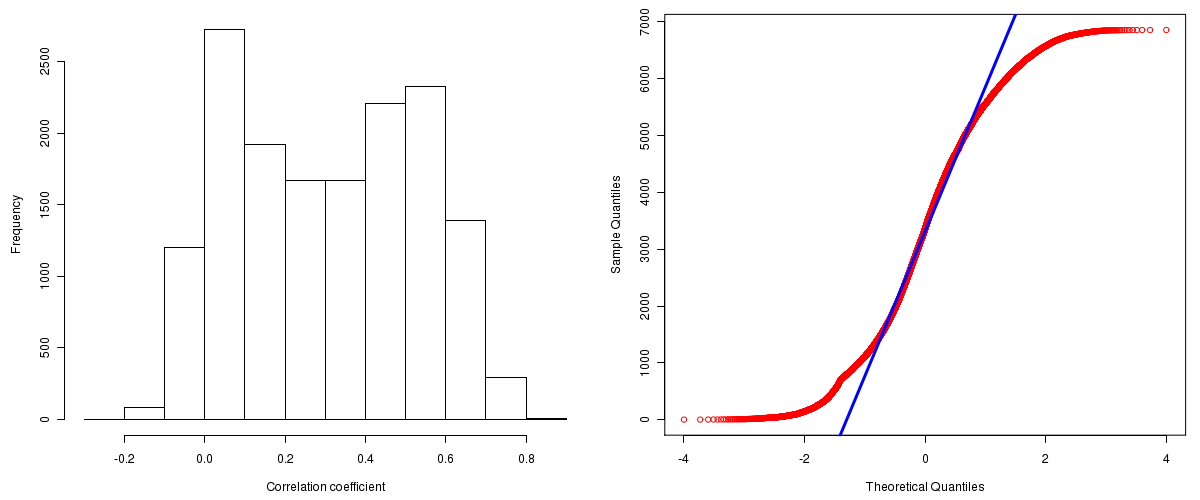Ovarian Serous Cystadenocarcinoma: Correlations between copy number and mRNA expression
Maintained by John Zhang (Dana-Farber Cancer Institute)
Overview
Introduction

A TCGA sample is profiled to detect the copy number variations and expressions of genes. This pipeline attempts to correlate copy number and expression data of genes across samples to determine if the copy number variations also result in differential expressions. This report contains the calculated correlation coefficients based on measurements of genomic copy number (log2) values and intensity of the expressions of the corresponding feature across patients. High positive/low negative correlation coefficients indicate that genomic alterations result in differences in the expressions of mRNA the genomic regions transcribe.

Summary

The correlation coefficients in 10, 20, 30, 40, 50, 60, 70, 80, 90 percentiles are 0.0114, 0.0651, 0.1292, 0.2158, 0.3097, 0.4016, 0.4715, 0.5399, 0.6072, respectively.

Results
Correlation results

Number of genes and samples used for the calculation are shown in Table 1. Figure 1 shows the distribution of calculated correlation coefficients and quantile-quantile plot of the calculated correlation coefficients against a normal distribution. Table 2 shows the top 20 features ordered by the value of correlation coefficients.

Table 1.  Counts of mRNA and number of samples in copy number and expression data sets and common to both

Category Copy number Expression Common
Sample 547 565 539
Genes 29390 18633 16124

Figure 1.  Summary figures. Left: histogram showing the distribution of the calculated correlations across samples for all Genes. Right: QQ plot of the calculated correlations across samples. The QQ plot is used to plot the quantiles of the calculated correlation coefficients against that derived from a normal distribution. Points deviating from the blue line indicate deviation from normality.Table 2.  Get Full Table Top 20 features (defined by the feature column) ranked by correlation coefficients

feature r p-value q-value chrom start end geneid
PAF1 0.8601 0 0 19 44568109 44573519 54623
TIMM50 0.8475 0 0 19 44662892 44673368 92609
C19orf12 0.8456 0 0 19 34883910 34897971 83636
C19orf2 0.8419 0 0 19 35125265 35198456 8725
TBP 0.8384 0 0 6 170705396 170723872 6908
GSTT1 0.8383 0 0 22 22706141 22714231 2952
ASCC2 0.8295 0 0 22 28514600 28564254 84164
ZNF7 0.8222 0 0 8 146023807 146039409 7553
ANKRD27 0.8048 0 0 19 37779755 37857919 84079
TYK2 0.8019 0 0 19 10322209 10352211 7297
C18orf8 0.799 0 0 18 19337471 19365740 29919
PSMC4 0.7974 0 0 19 45168913 45179193 5704
MBD1 0.7888 0 0 18 46049214 46061844 4152
RANBP3 0.7886 0 0 19 5867150 5929113 8498
STARD3 0.7874 0 0 17 35046938 35073263 10948
SUPT5H 0.7872 0 0 19 44628164 44659150 6829
UBE2L3 0.7869 0 0 22 20251957 20308323 7332
POP4 0.7864 0 0 19 34789041 34798547 10775
PSMF1 0.7859 0 0 20 1041939 1095971 9491
WBP11 0.7835 0 0 12 14830679 14847668 51729
Methods & Data
Input

Gene level (TCGA Level III) expression data and copy number data of the corresponding loci derived by using the CNTools package of Bioconductor were used for the calculations. Pearson correlation coefficients were calculated for each pair of genes shared by the two data sets across all the samples that were common.

Correlation across sample

Pairwise correlations between the log2 copy numbers and expressions of each gene across samples were calculated using Pearson correlation.